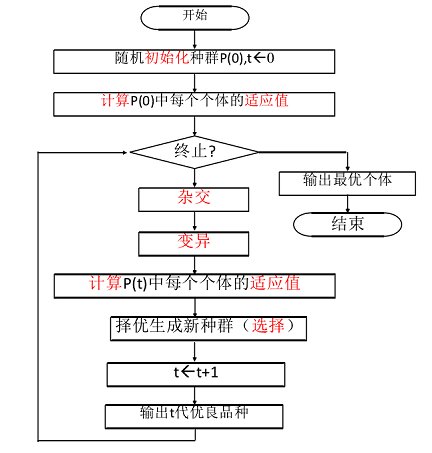# 自然选择的概念

## 核心步骤

1. 初始化

2. 个体评价（计算适应度函数）

3. 选择运算

4. 交叉运算

5. 变异运算

# 基本概念

## 交叉运算## 变异运算## 迭代的伪代码# java 实例

## 编码

public static final int GENG_LENGTH = 14;
private int x,y;
private String gene;


## 初始群体的产生

public Chromosome(String gene) {
this.gene = gene;
this.x = MathUtil.binaryStringToInt(gene.substring(0, gene.length() / 2));
this.y = MathUtil.binaryStringToInt(gene.substring(gene.length() / 2));
}

public Chromosome(int x, int y) {
this.x = x;
this.y = y;
//int 转二进制
this.gene = MathUtil.toBinaryString(x, 7) + MathUtil.toBinaryString(y, 7);
}


public static ArrayList<Chromosome> initGroup(int size) {
ArrayList<Chromosome> list = new ArrayList<Chromosome>();
Random random = new Random();
for(int i = 0; i < size; i++) {
int x = random.nextInt(128);
int y = random.nextInt(128);
}
return list;
}


## 适应度计算

ps: 如果是取最小值，可以取反之类的。（要考虑函数的结果符号）

public int calcFitness() {
return x*x+y*y;
}


## 选择运算

public static ArrayList<Chromosome> selector(ArrayList<Chromosome> fatherGroup,int sonGroupSize)
{
ArrayList<Chromosome> sonGroup = new ArrayList<Chromosome>();
int totalFitness = 0;
double[] fitness = new double[fatherGroup.size()];
for(Chromosome chrom : fatherGroup) {
totalFitness += chrom.calcFitness();
}
int index = 0;
//计算适应度
for(Chromosome chrom : fatherGroup) {
fitness[index] = chrom.calcFitness() / ((double)totalFitness);
index++;
}
//计算累加适应度
for(int i = 1; i < fitness.length; i++) {
fitness[i] = fitness[i-1]+fitness[i];
}
//轮盘赌选择
for(int i = 0; i < sonGroupSize; i++) {
Random random = new Random();
double probability = random.nextDouble();
int choose;
for(choose = 1; choose < fitness.length - 1; choose++) {
if(probability < fitness[choose])
break;
}
}
return sonGroup;
}
}


## 交叉运算

public static ArrayList<Chromosome> crossover(ArrayList<Chromosome> fatherGroup,double probability) {
ArrayList<Chromosome> sonGroup = new ArrayList<Chromosome>();
Random random = new Random();
for(int k = 0; k < fatherGroup.size() / 2; k++) {
if(probability > random.nextDouble()) {
int i = 0,j = 0;
// 为了保证不同
do {
i = random.nextInt(fatherGroup.size());
j = random.nextInt(fatherGroup.size());
} while(i == j);
int position = random.nextInt(Chromosome.GENG_LENGTH);

// 随机选择两个，进行基因的组合
String parent1 = fatherGroup.get(i).getGene();
String parent2 = fatherGroup.get(j).getGene();
String son1 = parent1.substring(0, position) + parent2.substring(position);
String son2 = parent2.substring(0, position) + parent1.substring(position);
}
}
return sonGroup;
}


ps: 这里是新增了两个子元素。

## 变异运算

public void selfMutation(String newGene) {
if(newGene.length() != Chromosome.GENG_LENGTH)
return;
this.gene = newGene;
String xStr = newGene.substring(0, Chromosome.GENG_LENGTH/2);
String yStr = newGene.substring(Chromosome.GENG_LENGTH/2);
this.x = Integer.parseInt(xStr,2);
this.y = Integer.parseInt(yStr,2);
}


public static void mutation(ArrayList<Chromosome> fatherGroup,double probability) {
Random random = new Random();
Chromosome bestOne = Chromosome.best(fatherGroup);
for(Chromosome c : fatherGroup) {
String newGene = c.getGene();
for(int i = 0; i < newGene.length();i++){
if(probability > random.nextDouble()) {
// 变异，基本反转
String newChar = newGene.charAt(i) == '0'?"1":"0";
newGene = newGene.substring(0, i) + newChar + newGene.substring(i+1);
}
}
c.selfMutation(newGene);
}
}


/**
* 最佳的个体
*
* @param fatherGroup 种群
* @return 最佳个体
*/
private static Chromosome best(ArrayList<Chromosome> fatherGroup) {
int bestFit = -1;
Chromosome best = null;
for (Chromosome chromosome : fatherGroup) {
int fitness = chromosome.calcFitness();
if (fitness > bestFit) {
bestFit = fitness;
best = chromosome;
}
}
return best;
}


## 测试效果

final int GROUP_SIZE = 20;//种群规模
final double MUTATION_P = 0.01;//变异概率
ArrayList<Chromosome> group = Chromosome.initGroup(GROUP_SIZE);
Chromosome theBest;

for(int i = 0; i < 180; i++) {
Chromosome.mutation(group, MUTATION_P);
group = Chromosome.selector(group, GROUP_SIZE);
System.out.println(Chromosome.best(group).calcFitness());
}


...
32005
32258
32258


# 拓展题目

求解 f=x*sin(10PI*x)+2 在 [0,4] 之间的最大值



# 参考资料

《演化程序-遗传算法和数据编码的结合》

《遗传算法与工程优化》

《Java 遗传算法编程》